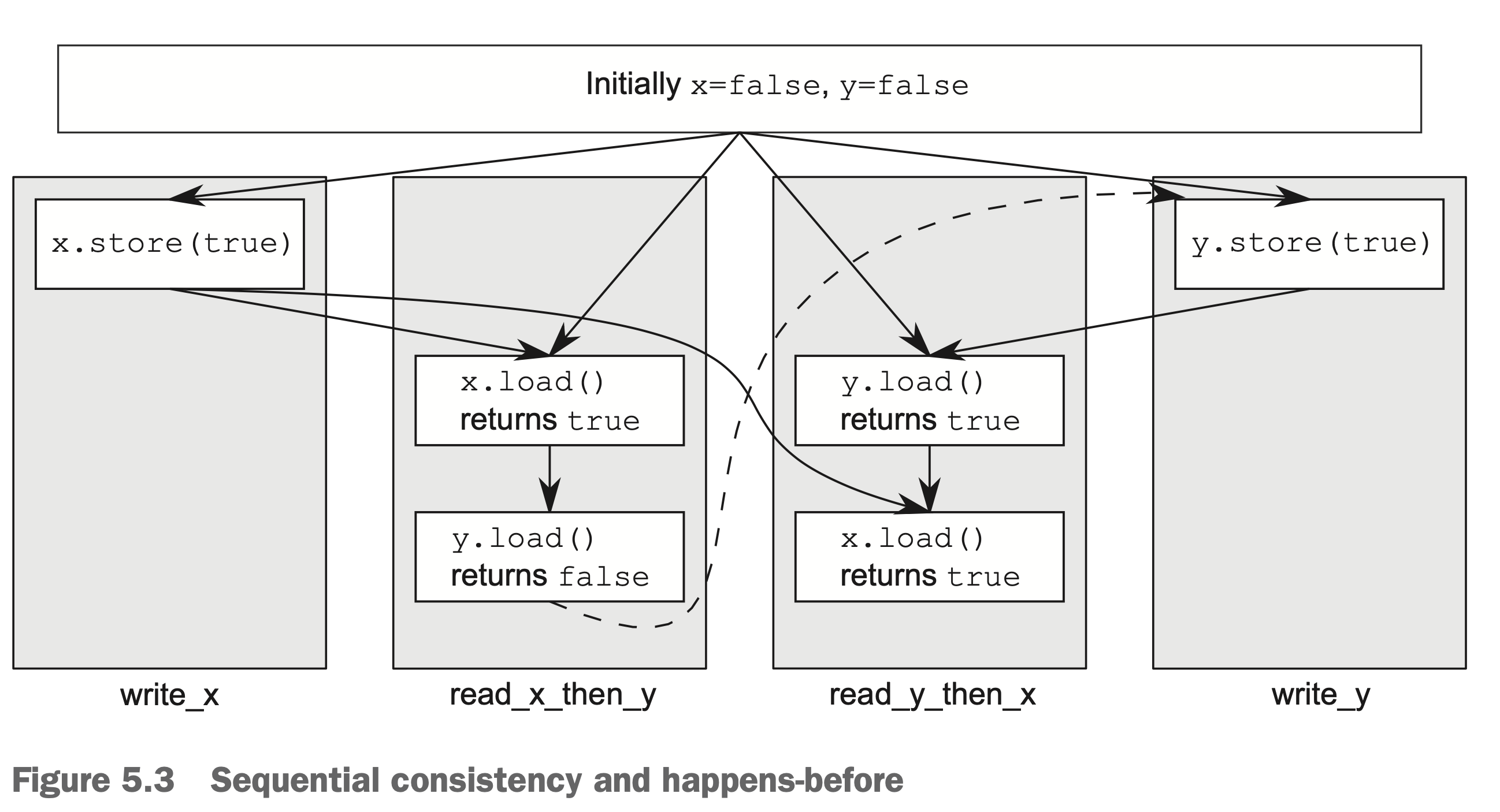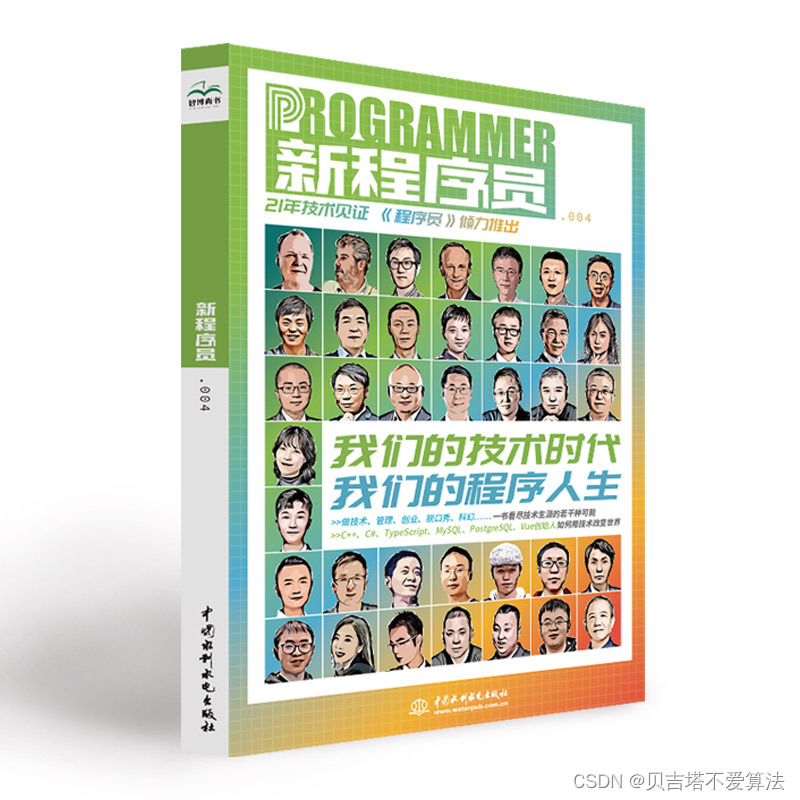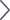> 文档中心 > C++11多线程 内存序(std::memory_order_seq_cst )

# C++11多线程 内存序(std::memory_order_seq_cst )

### C++11多线程内存序

• 内存模型
• 为什么要有内存模型
• C++11的内存模型种类
• 各个内存模型介绍及代码测试
• memory_order_seq_cst 顺序一致性模型

# 内存模型

## 为什么要有内存模型

### C++11的内存模型种类

C++11提供了6种内存序

``enum memory_order {    memory_order_relaxed,    memory_order_consume,    memory_order_acquire,    memory_order_release,    memory_order_acq_rel,    memory_order_seq_cst};``

# 各个内存模型介绍及代码测试

## memory_order_seq_cst 顺序一致性模型

``#include #include #include #include #include #include #include #include #include "ScopeThread.h"#include "template.h"#include "parallel_accumulate.h"#include "sequential_quick_sort.h"std::atomic<bool> x, y;std::atomic<int> z;void write_x() {    x.store(true, std::memory_order_seq_cst);}void write_y() {    y.store(true, std::memory_order_seq_cst);}void read_x_then_y() {    while (!x.load(std::memory_order_seq_cst)) {    }    if (y.load(std::memory_order_seq_cst)) { ++z;    }}void read_y_then_x() {    while (!y.load(std::memory_order_seq_cst));    if (x.load(std::memory_order_seq_cst)) { ++z;    }}int main() {    TimeSlap timeSlap;    x = false;    y = false;    z = 0;    std::thread a(write_x);    std::thread b(write_y);    std::thread c(read_x_then_y);    std::thread d(read_y_then_x);    a.join();    b.join();    c.join();    d.join();     for (int i = 0; i < 100000; ++i) { assert(z.load() != 0);    }    auto count_time = timeSlap.end();    std::cout << "消耗" << count_time << "秒" << std::endl;    return 0;}``

1. 首先随机选择一个线程b执行，此时y为true
2. 随机选择下一个线程a执行，此时x为true
3. 随机选择c线程执行，z为1
4. 随机选择d执行，z为2

1. 随机选择线程c执行，此时x为false，线程c一直循环
2. 随机选择线程d执行，此时y为fasle，线程d一直循环
3. 随机选择线程a执行，此时x为true
4. 随机选择线程c执行，此时y为false，x为true，z为0
5. 随机选择线程b执行，此时y为true，x为true
6. 随机选择线程d执行，此时z为1C++多线程 内存序(std::memory_order_relaxed)
C++多线程 内存序(std::memory_order_acquire/release)
C++多线程 内存序(std::memory_order_consume)
C++多线程 release sequence开发者涨薪指南48位大咖的思考法则、工作方式、逻辑体系